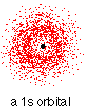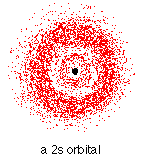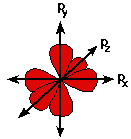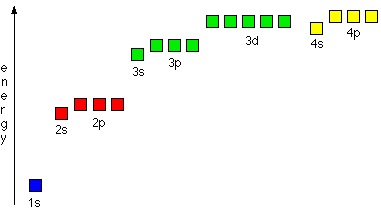Atomic Orbitals

•• Contributed by Jim Clark
• Former Head of Chemistry and Head of Science at Truro School in Cornwall

This page discusses atomic orbitals at an introductory level. It explores s and p orbitals in some detail, including their shapes and energies. d orbitals are described only in terms of their energy, and f orbitals are only mentioned in passing.

Orbitals and orbits

When a planet moves around the sun, its definite path, called an orbit, can be plotted. A drastically simplified view of the atom looks similar, in which the electrons orbit around the nucleus. The truth is different; electrons, in fact, inhabit regions of space known as orbitals. Orbits and orbitals sound similar, but they have quite different meanings. It is essential to understand the difference between them.

The impossibility of drawing orbits for electrons

To plot a path for something, the exact location and trajectory of the object must be known. This is imossible for electrons. The Heisenberg Uncertainty Principle states that it is impossible to define with absolute precision, at the same time, both the position and the momentum of an electron. That makes it impossible to plot an orbit for an electron around a nucleus.Figure 1: Hydrogen's electron - the 1s orbital

Consider a single hydrogen atom: at a particular instant, the position of the electron is plotted. The position is plotted again soon afterward, and it is in a different position. There is no way to tell how it moved from the first place to the second. This process is repeated many times, eventually creating a 3D map of the places that the electron is likely to be found.

In the hydrogen case, the electron can be found anywhere within a spherical space surrounding the nucleus. The figure above shows a cross-section of this spherical space. 95% of the time (or any arbitrary, high percentage), the electron is found within a fairly easily defined region of space quite close to the nucleus. Such a region of space is called an orbital, and it can be thought of as the region of space the electron inhabits. It is impossible to know what the electron is doing inside the orbital, so the electron's actions are ignored completely. All that can be said is that if an electron is in a particular orbital, it has a particular, definable energy.

Each orbital has a name.

The orbital occupied by the hydrogen electron is called a 1s orbital. The number "1" represents the fact that the orbital is in the energy level closest to the nucleus. The letter "s" indicates the shape of the orbital: s orbitals are spherically symmetric around the nucleus—​they look like hollow balls made of chunky material with the nucleus at the center.Figure 2: Hydrogen's electron - the 2s orbital

The orbital shown above is a 2s orbital. This is similar to a 1s orbital, except that the region where there is the greatest chance of finding the electron is further from the nucleus. This is an orbital at the second energy level. There is another region of slightly higher electron density (where the dots are thicker) nearer the nucleus ("electron density" is another way of describing the likelihood of an electron at a particular place).

2s (and 3s, 4s, etc.) electrons spend some of their time closer to the nucleus than might be expected. The effect of this is to slightly reduce the energy of electrons in s orbitals. The nearer the nucleus the electrons get, the lower their energy. 3s, 4s (etc.) orbitals are progressively further from the nucleus.

p orbitals

Not all electrons inhabit s orbitals (in fact, very few electrons live occupy s orbitals). At the first energy level, the only orbital available to electrons is the 1s orbital, but at the second level, as well as a 2s orbital, there are 2p orbitals. A p orbital is shaped like 2 identical balloons tied together at the nucleus. The orbital shows where there is a 95% chance of finding a particular electron.

Imagine a horizontal plane through the nucleus, with one lobe of the orbital above the plane and the other beneath it; there is a zero probability of finding the electron on that plane. How does the electron get from one lobe to the other if it can never pass through the plane of the nucleus? At an introductory level, it must simply be accepted. To find out more, read about the wave nature of electrons.

At any one energy level it is possible to have three absolutely equivalent p orbitals pointing mutually at right angles to each other. These are arbitrarily given the symbols px, py and pz. This is simply for convenience; the x, y, and z directions change constantly as the atom tumbles in space.Figure 3: Hydrogen's electron - the 2p orbitals

The p orbitals at the second energy level are called 2px, 2py and 2pz. There are similar orbitals at subsequent levels: 3px, 3py, 3pz, 4px, 4py, 4pz and so on. All levels except for the first level have p orbitals. At the higher levels the lobes are more elongated, with the most likely place to find the electron more distant from the nucleus.

d and f orbitals

In addition to s and p orbitals, there are two other sets of orbitals that become available for electrons to inhabit at higher energy levels. At the third level, there is a set of five d orbitals (with complicated shapes and names) as well as the 3s and 3p orbitals (3px, 3py, 3pz). At the third level there are nine total orbitals. At the fourth level, as well the 4s and 4p and 4d orbitals there are an additional seven f orbitals, adding up to 16 orbitals in all. s, p, d and f orbitals are then available at all higher energy levels as well.

Fitting electrons into orbitals

An atom can be pictured as a very strange house (somewhat an inverted pyramid), with the nucleus living on the ground floor, and then various rooms (orbitals) on the higher floors occupied by the electrons. On the first floor there is only 1 room (the 1s orbital); on the second floor there are 4 rooms (the 2s, 2px, 2py and 2pz orbitals); on the third floor there are 9 rooms (one 3s orbital, three 3p orbitals and five 3d orbitals); and so on. However, the rooms are not large: each orbital can only hold 2 electrons.

"Electrons-in-boxes"

A convenient way of showing the orbitals that the electrons live in is to draw "electrons-in-boxes". Orbitals can be represented as boxes with the electrons depicted with arrows. Often an up-arrow and a down-arrow are used to show that the electrons are different. The need for all electrons in an atom to be different originates from quantum theory. If the electrons inhabit different orbitals, they can have identical properties, but if they are both in the same orbital there must be a distinction between them. Quantum theory allocates them a property known as "spin," represented by the direction the arrow is pointing.

A 1s orbital holding 2 electrons is drawn as shown on the right, but it can be written even more quickly as 1s2. This is read as "one s two," not as "one s squared." Do not confuse the energy level with the number of electrons in this notation.The Aufbau Principle: the order of filling orbitals

Aufbau is a German word meaning building up or construction. Moving from one atom to the next in the periodic table, the electronic structure of the next atom can be determined by fitting an extra electron into the next available orbital. Electrons fill low energy orbitals (closer to the nucleus) before they fill higher energy ones. If there is a choice between orbitals of equal energy, they fill the orbitals singly as far as possible before pairing up.

This filling of orbitals singly where possible is known as Hund's rule. It only applies to orbitals with exactly the same energies (as with p orbitals, for example), and helps to minimize the repulsions between electrons and so makes the atom more stable. The diagram (not to scale) summarizes the energies of the orbitals up to the 4p level that you will need to know when you are using the Aufbau Principle.Notice that the s orbital always has a slightly lower energy than the p orbitals at the same energy level, so the s orbital always fills with electrons before the corresponding p orbitals do. The oddity is the position of the 3d orbitals. They are at a slightly higher level than the 4s, so the 4s orbital fills first, followed by all the 3d orbitals and then the 4p orbitals.